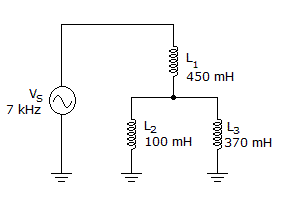# Electronics - Inductors - Discussion

### Discussion :: Inductors - Filling the Blanks (Q.No.3)

3.The inductive reactance of L2 is _____ in the given circuit.

 [A]. 3.5 k[B]. 4.4 k[C]. 16.3 k[D]. 23.3 kExplanation:

No answer description available for this question.

 Tim said: (Jun 25, 2014) Here total inductance is l = 1/l1+(1/(l2+l3)). l = 1/450mh+1/(100mh+370mh). l = (10^6/450+10^6/470) = (2.22222+2.12766)10^6. l = 4.34988k. Nearest value is 4.4 kohms.

 Just Me said: (Aug 24, 2014) If the Inductors were all in parallel your calculation makes sense. Just calculate the Inductive reactance for the 450mH inductor the value is 2*PI*7000*(450X 10^-3) = 19792 OHMs so the total reactance is higher than this value.

 Kiran V said: (Jan 25, 2017) XL = 2 * Pi * f * L. Given: L2 = 100 mH. XL = 2 * 3.14 * 7 * 10^3 * 100 * 10^-3. XL = 4396 Ohm. Nearly XL = 4.4 K Ohm.